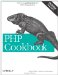# Recipe 1.16. Storing Binary Data in Strings

#### 1.16.1. Problem

You want to parse a string that contains values encoded as a binary structure or encode values into a string. For example, you want to store numbers in their binary representation instead of as sequences of ASCII characters.

#### 1.16.2. Solution

Use pack( ) to store binary data in a string:

`<?php \$packed = pack('S4',1974,106,28225,32725); ?>`

Use unpack( ) to extract binary data from a string:

`<?php \$nums = unpack('S4',\$packed); ?>`

#### 1.16.3. Discussion

The first argument to pack( ) is a format string that describes how to encode the data that's passed in the rest of the arguments. The format string S4 tells pack( ) to produce four unsigned short 16-bit numbers in machine byte order from its input data. Given 1974, 106, 28225, and 32725 as input on a little-endian machine, this returns eight bytes: 182, 7, 106, 0, 65, 110, 213, and 127. Each two-byte pair corresponds to one of the input numbers: 7 * 256 + 182 is 1974; 0 * 256 + 106 is 106; 110 * 256 + 65 = 28225; 127 * 256 + 213 = 32725.

The first argument to unpack( ) is also a format string, and the second argument is the data to decode. Passing a format string of S4, the eight-byte sequence that pack( ) produced returns a four-element array of the original numbers. print_r(\$nums) prints:

`Array (      => 1974      => 106      => 28225      => 32725 )`

In unpack( ), format characters and their count can be followed by a string to be used as an array key. For example:

`<?php \$nums = unpack('S4num',\$packed); print_r(\$nums); ?>`

This prints:

`Array (     [num1] => 1974     [num2] => 106     [num3] => 28225     [num4] => 32725 )`

Multiple format characters must be separated with / in unpack( ):

`<?php \$nums = unpack('S1a/S1b/S1c/S1d',\$packed); print_r(\$nums); ?> `

This prints:

`Array (     [a] => 1974     [b] => 106     [c] => 28225     [d] => 32725 )`

The format characters that can be used with pack( ) and unpack( ) are listed in Table 1-2.

##### Table 1-2. Format characters for pack( ) and unpack( )

Format character

Data type

a

A

h

Hex string, low nibble first

H

Hex string, high nibble first

c

signed char

C

unsigned char

s

signed short (16 bit, machine byte order)

S

unsigned short (16 bit, machine byte order)

n

unsigned short (16 bit, big endian byte order)

v

unsigned short (16 bit, little endian byte order)

i

signed int (machine-dependent size and byte order)

I

unsigned int (machine-dependent size and byte order)

l

signed long (32 bit, machine byte order)

L

unsigned long (32 bit, machine byte order)

N

unsigned long (32 bit, big endian byte order)

V

unsigned long (32 bit, little endian byte order)

f

float (machine-dependent size and representation)

d

double (machine-dependent size and representation)

x

NUL byte

X

Back up one byte

@

NUL-fill to absolute position

For a, A, h, and H, a number after the format character indicates how long the string is. For example, A25 means a 25-character space-padded string. For other format characters, a following number means how many of that type appear consecutively in a string. Use * to take the rest of the available data.

You can convert between data types with unpack( ). This example fills the array \$ascii with the ASCII values of each character in \$s:

`<?php \$s = 'platypus'; \$ascii = unpack('c*',\$s); print_r(\$ascii); ?>`

This prints:

`Array (      => 112      => 108      => 97      => 116      => 121      => 112      => 117      => 115 )`

Documentation on pack( ) at http://www.php.net/pack and unpack( ) at http://www.php.net/unpack .PHP Cookbook: Solutions and Examples for PHP Programmers
ISBN: 0596101015
EAN: 2147483647
Year: 2006
Pages: 445

Similar book on Amazon